International
Tables for
Crystallography
Volume B
Reciprocal space
Edited by U. Shmueli

International Tables for Crystallography (2010). Vol. B, ch. 2.5, pp. 368-369   | 1 | 2 |

## Section 2.5.6.3. Discretization and interpolation

B. K. Vainshteinc and P. A. Penczekg

#### 2.5.6.3. Discretization and interpolation

| top | pdf |

In digital image processing, space is represented by a multidimensional discrete lattice. (It is sometimes expedient to use cylindrical or spherical coordinates, but these also have to be appropriately discretized.) The 2D projections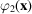are sampled on a Cartesian grid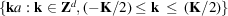, where d is the dimensionality of the grid (d = 2 for projections, d = 3 for the reconstructed object),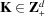is the size of the grid and a is the grid spacing (Fig. 2.5.6.4). In single-particle reconstruction, the units of a are usually ångstroms and we also assume that the data were appropriately sampled, i.e.,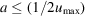. Thus, the pixel size is less than or equal to the inverse of twice the maximum spatial frequency present in the data. Since the latter is not known in advance, a more practical rule is to select the pixel size at about one third of the expected resolution of the final structure, so that the adverse effects of interpolation are reduced.Figure 2.5.6.4 | top | pdf |Discretization in two dimensions (d = 2). The assumption is that the mass is located at the centre of the voxel.

The input electron microscopy data (projections of the macromolecule) are discretized on a 2D Cartesian grid, but each projection has a particular orientation in polar coordinates. Except for a few cases (projection directions parallel to the axes of the coordinate system of the 3D structure), an interpolation is required to relate measured samples to the voxel (volume pixel) values on the 3D Cartesian grid of the reconstructed structure (Fig. 2.5.6.4). The step of backprojection can be visualized as a set of rays with base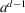extended from projections and the ray values being added to the intersected voxels on the grid of the reconstructed structure (Figs. 2.5.6.3and 2.5.6.4). One can select schemes that aim at approximation of the physical reality of the data collection, for example to weight the contributions by the areas of the voxels intersected by the ray or by the lengths of the lines that transverse the voxel (Huesman et al., 1977). In order to reduce the time of calculations, in electron microscopy one usually assumes that all the mass is located at the centre of the voxel and the additional accuracy is achieved by application of tri- (or bi-)linear interpolation. The exception is the algebraic reconstruction technique with blobs algorithm (Marabini et al., 1998), where the voxels are represented by smooth spherically symmetric volume elements [for example, the Keiser–Bessel function (2.5.6.44)].

In real space, both the projection and backprojection steps can be implemented in two different ways: as voxel driven or as ray driven (Laurette et al., 2000). If we consider a projection, in the voxel-driven approach the volume is scanned voxel by voxel. The nearest projection bin to the projection of each voxel is found, and the values in this bin and three neighbouring bins are increased by the corresponding voxel value multiplied by the weights calculated using bilinear interpolation. In the ray-driven approach, the volume is scanned along the projection rays. The value of the projection bin is increased by the values in the volume calculated in equidistant steps along the rays using trilinear interpolation. Because voxel- and ray-driven methods apply interpolation to projections or to voxels, respectively, the interpolation artifacts will be different in each case. Therefore, when calculating reconstructions using iterative algorithms that alternate between projection and backprojection steps, it is important to maintain consistency; that is, to use the same method for both steps. In either case, the computational complexity of each method is of the order of K3, although the voxel-driven approach is faster due to the smaller number of neighbouring points used in the interpolation.

In the reconstruction methods based on the direct Fourier inversion of the 3D ray transform, the interpolation is performed in Fourier space. Unfortunately, it is difficult to design an accurate and fast interpolation scheme for the discrete Fourier space. Bilinear interpolation introduces local errors and when applied in real space it results in attenuation of high-frequency information. When applied in Fourier space, bilinear interpolation results in errors evenly spread over the whole frequency range, thus resulting in potentially severe nonlocal errors in real space. In order to eliminate this error it would be tempting to use interpolation based on Shannon's sampling theorem [Shannon, 1949; reprinted in Proc. IEEE, (1998), 86, 447–457], which states that a properly sampled band-limited signal can be fully recovered from its discrete samples. For the signal represented by K3 equispaced Fourier samples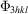, the value of the Fourier transform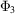at the arbitrary location (u, v, w) is given by (Crowther, Amos et al., 1970)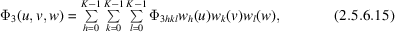where (Yuen & Fraser, 1979; Lanzavecchia & Bellon, 1994)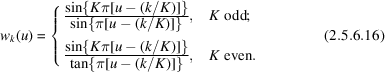In cryo-EM, samples ofare given at arbitrary 3D locations, as derived from Fourier transforms of 2D projection data (central section theorem) and one seeks to recoveron the 3D Cartesian grid. Upon the inverse Fourier transform, it will yield the reconstructed object. The problem can be solved as an overdetermined system of linear equations (Crowther, DeRosier & Klug, 1970). Indeed, if we writeandas 1D arrays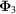and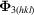, respectively (the former has K2 × [number of projections] elements, while the latter has K3 elements), and we denote by W the appropriately dimensioned matrix of the interpolants, the least-squares solution is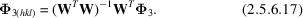The above method is impractical because of the large size of the matrix W. In some cases, when due to symmetries the projection data are distributed approximately evenly (as in the case of icosahedral structures), the problem can be solved to a good degree of accuracy by performing the interpolation (2.5.6.17)independently along each of the three frequency axes (Crowther, DeRosier & Klug, 1970). Thus, in this case the solution to the problem of interpolation in Fourier space becomes a solution to the reconstruction problem.

For a more general single-particle reconstruction application a moving Shannon window interpolation has been proposed (Lanzavecchia & Bellon, 1994, 1998). It is based on an attenuated version of the window function and in one dimension has the form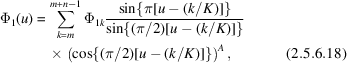where n is the window size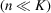and A is an integer that is even for K odd and vice versa. In multidimensional cases, a product of's from (2.5.6.18)is used. In the case of interpolation between equispaced samples of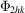, excellent results have been reported for n = 11 (Lanzavecchia et al., 1996). However, the application to the reconstruction problem, i.e., to resampling of the nonuniformly distributed Fourier data onto a 3D Cartesian grid, does not yield satisfactory results. Although general conditions under which interpolation using (2.5.6.18)can be done are known (Clark et al., 1985), they are not met in practice and the results are at best nonexact. In addition, the relatively large window size required (n = 11) results in impractical calculation times.

### References

Clark, J. J., Palmer, M. R. & Lawrence, P. D. (1985). A transformation method for the reconstruction of functions from nonuniformly spaced samples. IEEE Trans. Acoust. Speech Signal Process. 33, 1151–1165.
Crowther, R. A., Amos, L. A., Finch, J. T., DeRosier, D. J. & Klug, A. (1970). Three dimensional reconstruction of spherical viruses by Fourier synthesis from electron micrographs. Nature (London), 226, 421–425.
Crowther, R. A., DeRosier, D. J. & Klug, A. (1970). The reconstruction of a three-dimensional structure from projections and its application to electron microscopy. Proc. R. Soc. London Ser. A, 317, 319–340.
Huesman, R. H., Gullberg, G. T., Greenberg, W. L. & Budinger, T. F. (1977). RECLBL library users' manual – Donner algorithms for reconstruction tomography. University of California, Berkeley, USA.
Lanzavecchia, S. & Bellon, P. L. (1994). A moving window Shannon reconstruction for image interpolation. J. Visual Comm. Image Repres. 3, 255–264.
Lanzavecchia, S. & Bellon, P. L. (1998). Fast computation of 3D Radon transform via a direct Fourier method. Bioinformatics, 14, 212–216.
Lanzavecchia, S., Tosoni, L. & Bellon, P. L. (1996). Fast sinogram computation and the sinogram-based alignment of images. Comp. Appl. Bio. Sci. 12, 531–537.
Laurette, I., Zeng, G. L., Welch, A., Christian, P. E. & Gullberg, G. T. (2000). A three-dimensional ray-driven attenuation, scatter and geometric response correction technique for SPECT in inhomogeneous media. Phys. Med. Biol. 45, 3459–3480.
Marabini, R., Herman, G. T. & Carazo, J. M. (1998). 3D reconstruction in electron microscopy using ART with smooth spherically symmetric volume elements (blobs). Ultramicroscopy, 72, 53–65.
Shannon, C. E. (1949). Communication in the presence of noise. Proc. Inst. Radio Eng. 37, 10–21.
Yuen, C. K. & Fraser, D. (1979). Digital Spectral Analysis. Adelaide: CSIRO Pitman.